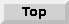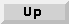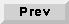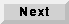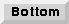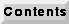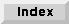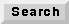2.4 Parameters and states

To see the parameter values of a star or galaxy, execute the Edit:edit-params command, which has the accelerator key "e". The singen star in the sinMod application has the following parameter screen: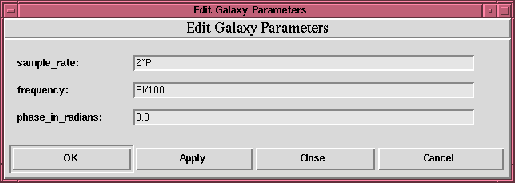2.4.1 A note on terminology

A State is a data-structure associated with a star and is used to remember data values from one invocation to the next. For example, the gain of an automatic gain control is a state. A state need not be dynamic since its value may not change during the course of a simulation. Technically, a parameter is the initial value of a state. Pigi is responsible for defining parameter values and storing them in the design database.

2.4.2 Changing or setting parameters

The edit-params command in pigi permits the user to set the initial value of a settable state of any star (lowest level block) and to define and set parameters for a galaxy (composite block) or universe (complete application).

Passing parameters through the hierarchy

Star parameters may be linked to the parameters of the galaxy or universe that contains the star. The syntax for linking the values of the star parameters to values of galaxy or universe parameters is simple. Consider again the sinMod application shown in figure 2-4. The parameter screen for the modulator block is shown below:This block, however, is a galaxy, not a star. If you look inside (as has been done in figure 2-4), and edit the parameters of the singen block inside modulator, you will see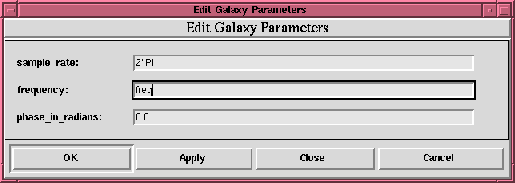Notice now that the value of the frequency parameter is a symbolic expression, "freq". This refers to the galaxy parameter "freq". Thus, parameter values can be passed down through the hierarchy. These symbolic references can appear in expressions, which we discuss next.

Parameter expressions

Parameter values set through pigi can be arithmetic expressions. This is particularly useful for propagating values down from a universe parameter to star parameters somewhere down in the hierarchy. An example of a valid parameter expression is:

PI/(2*order)
where order is a parameter defined in the galaxy or universe. The basic arithmetic operators are addition (+), subtraction (-), multiplication (*), division (/), and exponentiation (^). These operators work on integers and floating-point numbers. Currently all intermediate expressions are converted to the type of the parameter being computed. Hence, it is necessary to be very careful when, for example, using floating-point values to compute an integer parameter. In an integer parameter specification, all intermediate expressions will be converted to integers.

Complex-valued parameters

When defining complex values, the basic syntax is

(real, imag)
where real and imag evaluate to integers or floats.

Fixed-point parameters

Fixed-point parameters may be assigned a precision directly. To do this, the parameter is given in the syntax "(value, precision)", where value is an ordinary number and precision is given by either of two syntaxes:

In both cases, the sign bit counts as one of the integer bits, so this number must be at least one.

2.4.3 Reading Parameter Values From Files

The values of most parameter types can be read from a file. This syntax for this is to use the symbol < as in the following example:

< filename
First, any parameters appearing in the filename in the form of {parameter} are replaced with their values. Then, any references to environment variables or home directories are substituted to generate a complete path name. Finally, the contents of the file are then read and spliced into the parameter expression and reparsed. File inputs can be very useful for array parameters which may require a large amount of data. Other expression may come before or after the <filename syntax (any white space that appears after the < character is ignored).

Comments are also supported for non-string parameters. A comment is specified with the # symbol. Everything after the # until the end of the line is discarded when the parameter is evaluated. Comments are especially useful in combination with files as they can help remind the user of which galaxy or star parameter the file was written.

freq # This is set to the Galaxy parameter
Comments are not supported for the String parameter or String Array parameter types. In fact, when the image processing stars use String states to represent a filename, the # character is used to denote the frame number of the image being processed.

2.4.5 Using Tcl Expressions in Parameters

Arbitrary Tcl expressions can be embedded in a parameter expression by preceding the expression with the ! character as in the following example:

! "expression"
First, parameters in the form of {parameter} appearing in the expression are replaced by their values. Then, the string is sent to the pigiRpc Tcl interpreter for evaluation. Finally, the result is spliced into the parameter expression and reparsed. The pigiRpc Tcl interpreter is the same interpreter that appears as a window when pigi is started by using pigi -console.

! "expr sqrt(2.0 / {BitDuration})"
for the amplitude of the oscillators in a binary frequency shift keying system, in which BitDuration is a parameter. The expr command is a Tcl command that treats its arguments as a single mathematical expression that must evaluate to a number.

! "join 2*gain1"
Because gain1 is not surrounded by curly braces, its value is not substituted before passing the expression to the Tcl interpreter. The Tcl interpreter will return 2*gain1 which is then evaluated by the parameter parser.

!"list /users/ptolemy/myfile"
There are several Tcl commands embedded in pigiRpc that help support parameter calculations. They are: listApplyExpression, max, min, range, rangeApplyExpression, and sign. For example,

! "min [max 1 2 3] [sign -2]"
first evaluates to min 3 -1 and then to -1. The procedure range returns a consecutive sequence of numbers:

! "range 0 5"
returns 0 1 2 3 4 5. The rangeApplyExpression procedure generates a sequence of values by applying a consecutive sequence of numbers to a Tcl expression that is a function of i. For example, you can generate the taps of an FIR filter that is a sampled sinusoid by using

! "rangeApplyExpression { cos(2*{PI}*\$i/5) } 0 4"
generates one period of sinusoidal function and returns

1.0 0.309042 -0.808986 -0.809064 0.308916
The listApplyExpression is similar to rangeApplyExpression except that it only takes two arguments: the second argument is a list of numbers to substitute for i in the expression. The command

! "listApplyExpression { cos(2*{PI}*\$i/5) } [range 0 4]"
is equivalent to the previous example of the rangeApplyExpression function.

help sign
at the prompt. To start Tycho from within pigi, type a y while the mouse is over a vem facet or palette.

2.4.6 Using Matlab and Mathematica to Compute Parameters

Since Tcl can be used to compute parameters as described in the previous section, Ptolemy's Tcl interface to Matlab [Han96] and Mathematica [Wol91][Bla92] can be used to compute parameters. This allows even more expressiveness, but the drawback is that demonstrations relying on Matlab and Mathematica will only work at sites that have Matlab and Mathematica installed. For example, we can use Matlab to design an 32-order FIR half-band filter using the Parks-McClellan optimal equiripple FIR filter design algorithm:

! "matlab getpairs c {c=remez(32, [0 0.4 0.6 1], [1 1 0 0])}"
Similarly, we can use Mathematica to derive formulas to be used as parameters:

! "mathematica get c {c=Integrate[A x, {x, 0, 1}]}"
This command returns the symbolic expression A/2 which is reparsed by Ptolemy. Matlab and Mathematica can be used to keep track of how parameter values are computed. Mathematica can also be used to return symbolic expressions that can be used in conjunction with higher-order functions to define scalable systems [Eva95].

2.4.7 Array parameters

When defining arrays of integers, floats, complex numbers, fixed-point numbers, or strings, the basic syntax is a simple list separated by spaces. For example,

1 2 3 4 5
defines an integer array with five elements. The elements can be expressions if they are surrounded by parentheses:

1 2 PI (2*PI)
Repetition can be indicated using the following syntax:

value[n]
where n evaluates to an integer. An array or portion of an array can be input from a file using the symbol < as in the following example:

1 2 < filename 3 4
Here the first two elements of the array will be 1 and 2, the next elements will be read from file filename, and the last two elements will be 3 and 4. This latter capability can be used in combination with the WaveForm star to read a signal from a file.

2.4.8 String Parameters

There is a bit of complication when one wishes to set a string parameter or string array parameter equal to the value of a galaxy or universe parameter. This is because a distinction must be made between a sequence of characters that give the name of a symbol and a sequence of characters to be interpreted literally. The syntax to use is explained in the example:

This string has the word {word} taken from another parameter
Here {word} represents the value of a string universe or galaxy parameter. This capability is especially useful for constructing labels for output plots. When using string states to specify options for a Unix command, as in the options parameter in Xgraph stars, you can use either double quotes or single quotes to include white space within a single word:

-0 'original signal' -1 'estimated signal'
String arrays have a few more special restrictions. Each word (separated by white space) is a separate entry in the array. To include white space in an element of the array, use quotation marks. Thus, the following string array

first "the second element" third
has three elements in it. The string array

repeat
has ten separate copies of the string "repeat" in 10 separate entries in the array. Curly braces are used to substitute in values from galaxy parameters. Thus, in

{paramname}
paramname must be the name of either a string array or a scalar-valued parameter (an integer, float or complex array, for example, is not permitted). If it is a string array, then each element of paramname becomes an element of the parameter. If it is some other kind of parameter the value becomes a single element of the string array.

String array values may also be read from files using the < symbol. For details on how to use file references, see section 2.4.3 above. Note that for string arrays, the filename can be a
literal string such as

< \$PTOLEMY/data/filename
as well as a string that refers to parameters such as

< \$PTOLEMY/{data_dir}/data_file
in which case the value of the parameter data_dir would be substituted. Ptolemy does not perform expansion of filenames such as file.{1,2} into file1 file2 as a Unix shell might do.